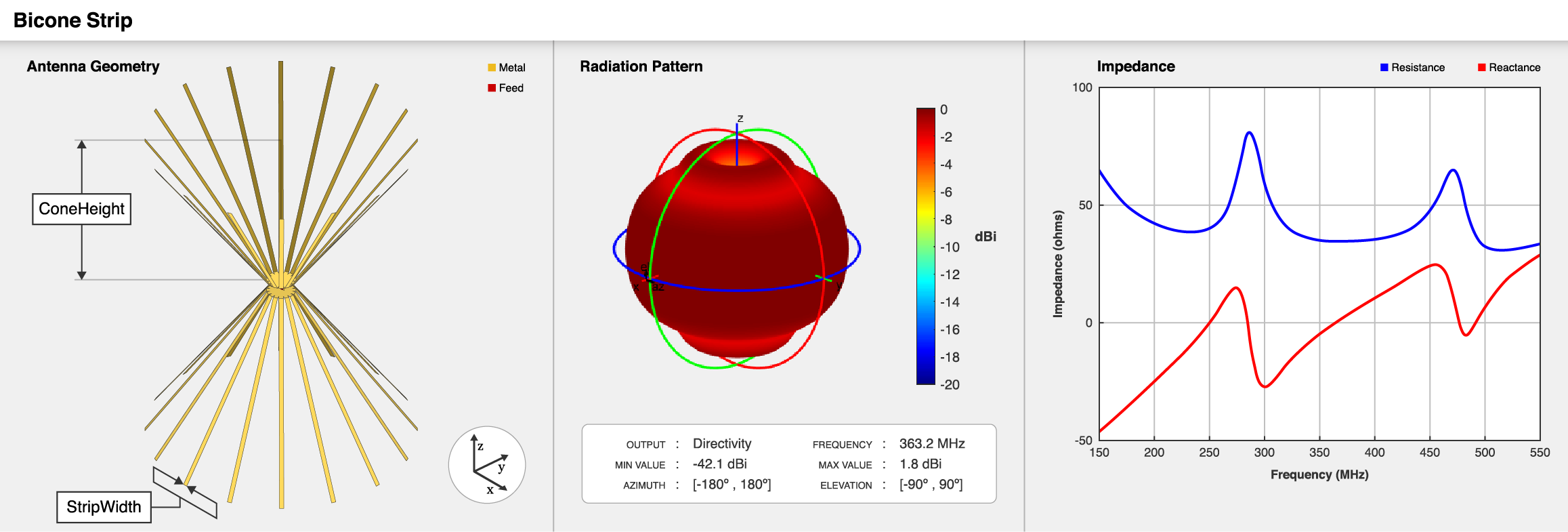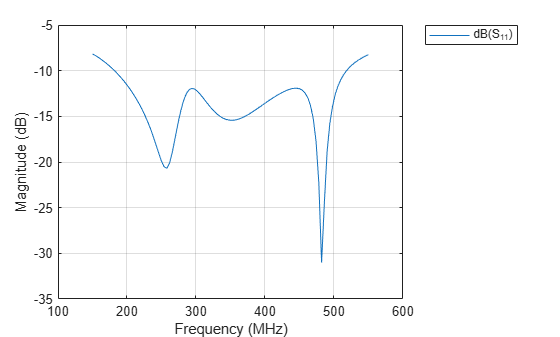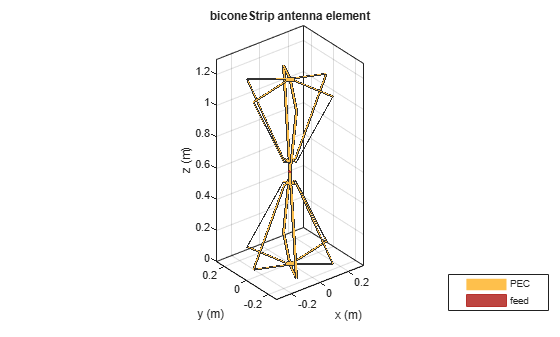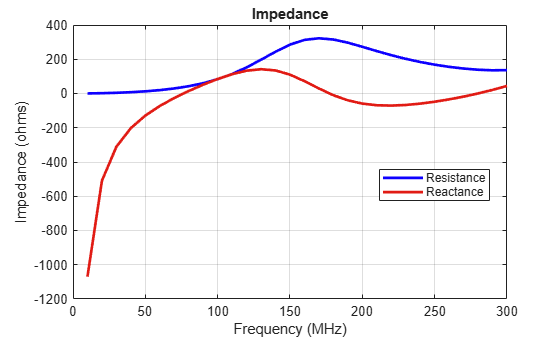# biconeStrip

Create strip bicone antenna

## Description

The `biconeStrip` object creates a strip bicone antenna. The strip bicone antenna is an approximation of a solid bicone antenna, where strips are used to approximate the two cones. The strip configuration makes these antennas lightweight and reduces wind loading. These antennas are more suitable for use at low frequencies. Strip bicone antennas are popular for their wide-impedance bandwidth and omnidirectional radiation coverage. These antennas are used in applications like emission testing, field monitoring, and chamber characterization.There are two types of bicone strip antennas, open-ended and phantom biconical. Specify the `HatHeight` property to create a phantom strip bicone antenna.

## Creation

### Syntax

``ant = biconeStrip``
``ant = biconeStrip(Name,Value)``

### Description

example

````ant = biconeStrip` creates a strip bicone antenna with dimensions for a resonant frequency of 363.2 MHz. ```

example

````ant = biconeStrip(Name,Value)` sets `Properties` using one or more name-value pairs. For example, ```ant = biconeStrip('NumStrips', 8)``` creates a strip bicone antenna with eight strips.```

## Properties

expand all

Number of strips to form the two cones of strip bicone antenna, specified a scalar integer in the range `[6,64]`.

Example: `'NumStrips',8`

Example: `ant.NumStrips = 8`

Data Types: `double`

Width of each strip, specified as positive scalar in meters.

Example: `'StripWidth',0.02`

Example: `ant.StripWidth = 0.02`

Data Types: `double`

Vertical height of the two hats, specified as either of the following:

• `0`— This creates open-ended strip bicone antenna.

• Positive scalar in meters— This creates two cone hats of same height.

• Two-element vector with each element unit in meters— This creates two cone hats of different heights. In the two-element vector, the first element specifies the hat height of the top cone, and the second element specifies the hat height of the bottom cone.

Example: `'HatHeight',0.045`

Example: `ant.HatHeight = 0.045`

Data Types: `double`

Vertical height of the two cones, specified as either of the following:

• Positive scalar in meters: This creates two cones of same height.

• Two-element vector with each element unit in meters: This creates two cones of different heights. In the two-element vector, the first element specifies the height of the top cone, and the second element specifies the height of the bottom cone.

Example: `'ConeHeight',0.5`

Example: `ant.ConeHeight = 0.5`

Data Types: `double`

Radius at the apex of the cones, specified as either of the following:

• Positive scalar in meters: This creates two cones with the same narrow radius.

• Two-element vector with each element unit in meters: This creates two cones with different narrow radii. In the two-element vector, the first element specifies the narrow radius of the top cone, and the second element specifies the narrow radius of the bottom cone.

Example: `'NarrowRadius',0.04`

Example: `ant.NarrowRadius = 0.04`

Data Types: `double`

Radius at the broad opening of the cones, specified as either of the following:

• Positive scalar in meters: This creates two cones with the same broad radius.

• Two-element vector with each element unit in meters: This creates two cones with different broad radii. In the two-element vector, the first element specifies the broad radius of the top cone, and the second element specifies the broad radius of the bottom cone.

Example: `'BroadRadius',0.7`

Example: `ant.BroadRadius = 0.7`

Data Types: `double`

Height of the feed spanning the gap between the two cones, specified as positive scalar in meters.

Example: `'FeedHeight',0.04`

Example: `ant.FeedHeight = 0.04`

Data Types: `double`

Width of the feed of the antenna, specified as a positive scalar in meters.

Example: `'FeedWidth',0.03`

Example: `ant.FeedWidth = 0.03`

Data Types: `double`

Type of the metal used as a conductor, specified as a metal material object. You can choose any metal from the `MetalCatalog` or specify a metal of your choice. For more information, see `metal`. For more information on metal conductor meshing, see Meshing.

Example: `m = metal('Copper'); 'Conductor',m`

Example: `m = metal('Copper'); ant.Conductor = m`

Tilt angle of the antenna, specified as a scalar or vector with each element unit in degrees. For more information, see Rotate Antennas and Arrays.

Example: `'Tilt',90`

Example: `ant.Tilt = 90`

Example: `'Tilt',[90 90]`,```'TiltAxis',[0 1 0;0 1 1]``` tilts the antenna at 90 degrees about the two axes defined by the vectors.

Data Types: `double`

Tilt axis of the antenna, specified as:

• Three-element vector of Cartesian coordinates in meters. In this case, each coordinate in the vector starts at the origin and lies along the specified points on the x-, y-, and z-axes.

• Two points in space, each specified as three-element vectors of Cartesian coordinates. In this case, the antenna rotates around the line joining the two points in space.

• A string input describing simple rotations around one of the principal axes, 'x', 'y', or 'z'.

Example: `'TiltAxis',[0 1 0]`

Example: `'TiltAxis',[0 0 0;0 1 0]`

Example: `ant.TiltAxis = 'Z'`

Lumped elements added to the antenna feed, specified as a `lumpedElement` object. You can add a load anywhere on the surface of the antenna. By default, the load is at the feed. For more information, see `lumpedElement`.

Example: `'Load',lumpedElement`. `lumpedElement` is the object for the load created using `lumpedElement`.

Example: `ant.Load = lumpedElement('Impedance',75)`

## Object Functions

 `coneangle2size` Calculates equivalent cone height, broad radius, and narrow radius for cone `show` Display antenna or array structure; display shape as filled patch `axialRatio` Axial ratio of antenna `beamwidth` Beamwidth of antenna `charge` Charge distribution on metal or dielectric antenna or array surface `current` Current distribution on metal or dielectric antenna or array surface `design` Design prototype antenna or arrays for resonance around specified frequency `efficiency` Radiation efficiency of antenna `EHfields` Electric and magnetic fields of antennas; Embedded electric and magnetic fields of antenna element in arrays `impedance` Input impedance of antenna; scan impedance of array `mesh` Mesh properties of metal or dielectric antenna or array structure `meshconfig` Change mesh mode of antenna structure `optimize` Optimize antenna or array using SADEA optimizer `pattern` Radiation pattern and phase of antenna or array; Embedded pattern of antenna element in array `patternAzimuth` Azimuth pattern of antenna or array `patternElevation` Elevation pattern of antenna or array `rcs` Calculate and plot radar cross section (RCS) of platform, antenna, or array `returnLoss` Return loss of antenna; scan return loss of array `sparameters` Calculate S-parameter for antenna and antenna array objects `vswr` Voltage standing wave ratio of antenna

## Examples

collapse all

Create a strip bicone antenna with default properties.

`ant = biconeStrip`
```ant = biconeStrip with properties: NumStrips: 16 StripWidth: 0.0180 HatHeight: 0 ConeHeight: 0.6650 NarrowRadius: 0.0700 BroadRadius: 0.6470 FeedHeight: 0.0450 FeedWidth: 0.0400 Conductor: [1x1 metal] Tilt: 0 TiltAxis: [1 0 0] Load: [1x1 lumpedElement] ```

View the antenna using the `show` function.

`show(ant);`Plot the S-parameters of the antenna over the frequency span of 150-550 MHz.

```s = sparameters(ant,linspace(150e6,550e6,101)); rfplot(s)```Create a strip bicone antenna with hat.

```ant = biconeStrip("NumStrips",6,"StripWidth",12e-3,"HatHeight",53e-3, ... "ConeHeight",465e-3,"NarrowRadius",40e-3,"BroadRadius",257e-3, ... "FeedHeight",144e-3,"FeedWidth",25e-3);```

View the antenna using the `show` function.

`show(ant)`Calculate antenna impedance over the frequency span of 10-300 MHz.

`impedance(ant,10e6:10e6:300e6)`